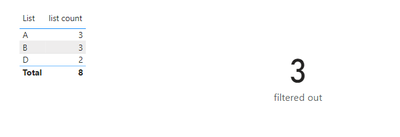cancel
Showing results for
Did you mean:

Fabric is Generally Available. Browse Fabric Presentations. Work towards your Fabric certification with the Cloud Skills Challenge.Frequent Visitor

## Distinct count when count of elements are higher than

hello everyone,

I'm trying to do the following with no useful result.

I have to get the list of unique names and their numbers from a column in a table. Furthermore, i have to calculate the total number of the overall list.  However, the list should consider only the values that are repeated for a number of times greater than x (for example 1)

For example:

 A A A B B B C D D

This should result in a list made of:

 A 3 B 3 D 2

And a total distinct count measure of 8, because we don't consider "C"

How can i do this? I know of the distinct and distinctcount functions, but i'm not able to filter my table in the correct way.

Sorry but i'm new to PBI and i cant figure it out.

Thank you all for the help

1 ACCEPTED SOLUTIONCommunity Support

Hi @Andrylodra ,

According to your description, here are my steps you can follow as a solution.

(1) My test data is the same as yours.

(2) We can create measures.

``````Measure =
var _a=CALCULATE(MAX('Table'[List]),FILTER('Table',COUNT('Table'[List])>1))
var _b= DISTINCT('Table'[List])
return IF(_a in _b ,1,0)``````
``list count = COUNTROWS(FILTER('Table','Table'[Measure]=1))``
``filtered out = CALCULATE(DISTINCTCOUNT('Table'[List]),FILTER(ALLSELECTED('Table'),[list count]<>BLANK()))``

(3) Then the result is as follows.Best Regards,

Neeko Tang

If this post  helps, then please consider Accept it as the solution  to help the other members find it more quickly.

3 REPLIES 3Community Support

Hi @Andrylodra ,

According to your description, here are my steps you can follow as a solution.

(1) My test data is the same as yours.

(2) We can create measures.

``````Measure =
var _a=CALCULATE(MAX('Table'[List]),FILTER('Table',COUNT('Table'[List])>1))
var _b= DISTINCT('Table'[List])
return IF(_a in _b ,1,0)``````
``list count = COUNTROWS(FILTER('Table','Table'[Measure]=1))``
``filtered out = CALCULATE(DISTINCTCOUNT('Table'[List]),FILTER(ALLSELECTED('Table'),[list count]<>BLANK()))``

(3) Then the result is as follows.Best Regards,

Neeko Tang

If this post  helps, then please consider Accept it as the solution  to help the other members find it more quickly.Solution Sage

Hope the following helps:Frequent Visitor

This solves a part of the problem, thanks.

Is there a way to count how many names were filtered out (in this case 3, because we have A,B and D)?

I can't use distinctcount on a measure if I'm correctAnnouncements#### Power BI Monthly Update - November 2023

Check out the November 2023 Power BI update to learn about new features.#### The largest Power BI and Fabric virtual conference

130+ sessions, 130+ speakers, Product managers, MVPs, and experts. All about Power BI and Fabric. Attend online or watch the recordings.Top Solution Authors
Top Kudoed Authors
Users online (5,720)